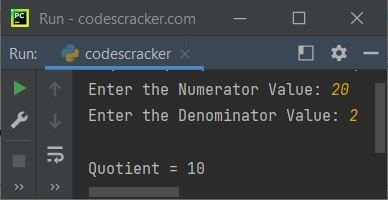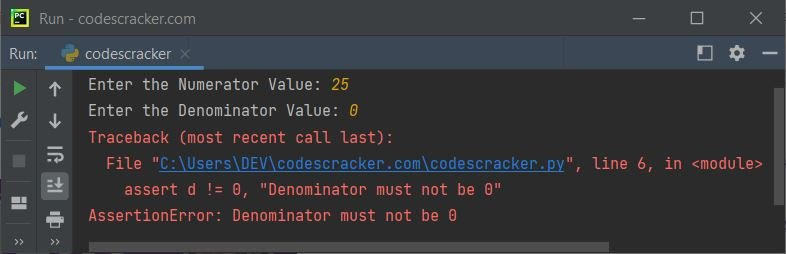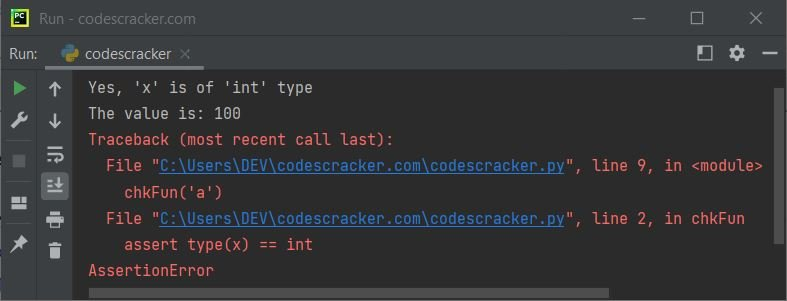# Python assert Keyword, assert Statement

The assert keyword in Python is used when we need to detect problems early, in a program where the cause is clear. For example:

```print("Enter the Numerator Value: ", end="")
num = int(input())
print("Enter the Denominator Value: ", end="")
den = int(input())

assert den != 0

quot = int(num/den)
print("\nQuotient =", quot)```

The snapshot given below shows the sample run with user input 20 as numerator and 2 as denominator:Following is another sample run, with user input 14 as numerator and 0 as denominator:Because the den is 0, therefore the assert's condition evaluates as False. Therefore, the program execution stopped, and thrown the AssertionError on output.

Note - The assert keyword is used, basically as a debugging purpose, as it halts the program, when an error occurs.

The above program can also be created in this way:

```print("Enter the Numerator Value: ", end="")
n = int(input())
print("Enter the Denominator Value: ", end="")
d = int(input())

if not d != 0:
raise AssertionError()

print("\nQuotient =", int(n/d))```

You'll get the similar output as of that program.

## Python assert Statement Syntax

The syntax of assert statement in Python, is:

`assert condition`

or

`assert condition, error_message`

In the second syntax, we can also use error_message to display the error. For example:

```print("Enter the Numerator Value: ", end="")
n = int(input())
print("Enter the Denominator Value: ", end="")
d = int(input())

assert d != 0, "Denominator must not be 0"

print("\nQuotient =", int(n/d))```

The sample run with user input 25 as numerator and 0 as denominator, is shown in the snapshot given below:## Python assert Keyword Example

Here is an example of assert statement or keyword in Python:

```def chkFun(x):
assert type(x) == int
print("Yes, 'x' is of 'int' type")
print("The value is:", x)

chkFun(100)

chkFun('a')

chkFun(10)```

The snapshot given below shows its sample output:That is, inside the function named chkFun(), the passed parameter 100 for first time, is of int type, therefore the type(x) == int evaluates to be True, and the two print() statements gets executed.

But for the second call of chkFun() function, the passed parameter 'a' is not of int type, therefore type(x) == int evaluates to be False, and the program halt, with AssertionError

Python Online Test

« Previous Tutorial Python Built-in Methods »